JOURNAL OFSYNCHROTRONRADIATION
ISSN: 1600-5775
Volume 20| Part 1| January 2013| Pages 49-57

Optimizing the spatial distribution of dose in X-ray macromolecular crystallography

aLaboratory of Molecular Biophysics, Department of Biochemistry, University of Oxford, South Parks Road, Oxford OX1 3QU, UK
*Correspondence e-mail: elspeth.garman@bioch.ox.ac.uk

(Received 27 July 2012; accepted 29 October 2012; online 29 November 2012)

X-ray data collection for macromolecular crystallography can lead to highly inhomogeneous distributions of dose within the crystal volume for cases when the crystal is larger than the beam or when the beam is non-uniform (Gaussian-like), particularly when crystal rotation is fully taken into account. Here the spatial distribution of dose is quantitatively modelled in order to compare the effectiveness of two dose-spreading data-collection protocols: helical scanning and translational collection. Their effectiveness in reducing the peak dose per unit diffraction is investigated via simulations for four common crystal shapes (cube, plate, long and short needles) and beams with a wide range of full width half maximum values. By inspection of the chosen metric, it is concluded that the optimum strategy is always to use as flat (top-hat) a beam as possible and to either match the beam size in both dimensions to the crystal, or to perform a helical scan with a beam which is narrow along the rotation axis and matched to the crystal size along the perpendicular axis. For crystal shapes where this is not possible, the reduction in peak dose per unit diffraction achieved through dose spreading is quantified and tabulated as a reference for experimenters.

1. Introduction

Radiation damage is widely recognized as one of the key bottlenecks in macromolecular structure solution by crystallography at both room temperature and 100 K (Garman, 2010; Holton, 2009). There are two main parameters that affect the extent of radiation damage in a crystal: the absorption sensitivity of the crystal to incident photons, and how many photons are incident on the crystal. These are considered together in terms of the dose, the amount of energy absorbed per unit mass of crystal (Gy = J kg−1). The dose absorbed by a crystal fully immersed in a top-hat beam (uniform intensity) is given by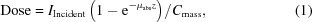where IIncident is the total incident energy flux on the crystal, and μabs, z and Cmass are the absorption coefficient, the thickness and the total irradiated mass of the crystal, respectively. The sensitivity of the crystal to damage is pivotally affected by the absorption coefficient, itself a function of the crystal composition and beam energy. The physical origin of this and how it is calculated is well described in previous work (Murray et al., 2005). Strategies to minimize the crystal sensitivity through the selection of weakly absorbing cryobuffers and mother liquors are emerging (Murray et al., 2004; Holton, 2009). Work on the effect of free-radical scavengers on the radiation sensitivity of crystals has shown some promise [see Allan et al. (2013) for a survey], but, since there are still conflicting results and many unresolved questions, the field remains very active. A significant factor in the conflicting results from radiation studies in general is the natural in­homogeneity of protein crystals grown in the same drop and given nominally the same treatment (e.g. Nowak et al., 2009).

The software program RADDOSE (Murray et al., 2004; Paithankar et al., 2009; Paithankar & Garman, 2010) is widely used to calculate the absorbed dose for a macromolecular crystal. It requires the beam properties (size, profile in x and y, flux and energy), the unit-cell parameters, the number of amino acids in the protein, and the mother-liquor/cryobuffer constituents as inputs, and uses them to estimate the overall absorption coefficient of the crystal. This calculation takes into account all physical phenomena relevant in the incident X-ray energy ranges used in macromolecular crystallography (MX) (7–20 keV or 1.6–0.56 Å): the photoelectric effect in RADDOSE V1 (version 1) (Murray et al., 2004), as well as fluorescence escape after photoelectric absorption in RADDOSE V2 (Paithankar et al., 2009) and the Compton effect in RADDOSE V3 (Paithankar & Garman, 2010).

As described in previous work (Garman, 2010), for a typical 100 µm-thick macromolecular crystal uniformly irradiated by 12.4 keV photons (1 Å), only 2% of the incident photons will interact with the crystal (estimated from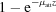with z = 100 µm, where μatt is the attenuation coefficient of the beam in the crystal). Under these conditions, of the 2% of interacting photons, 84% undergo photoelectric absorption, 8% are subject to the Compton effect and 8% are Rayleigh scattered (elastic, so interfere coherently to give diffraction). Some fraction (dependent on the atomic number of the element) of the photoelectrically absorbed photons will lead to fluorescence (as opposed to emission of an Auger electron), and there is a probability of these fluorescent photons escaping the crystal without further interactions, leading to a lower effective dose absorbed by the crystal. An estimation of the fraction of energy originating from the photoelectric effect escaping from the crystal was introduced in the update from RADDOSE V1 to V2. Fluorescence is only significant for heavier elements: e.g. for an iron atom there is a 34% probability of fluorescence production as opposed to emission of an Auger electron.

For the simple geometrical case described above (uniform X-ray exposure throughout the crystal volume), the RADDOSE treatment is accurate and offers robust guidance regarding the uniform dose (30 MGy) that a crystal can withstand before biological fidelity is compromised at 100 K (Owen et al., 2006) if no electron scavengers are present (Allan et al., 2013). Diffraction from the crystal may not be sustained until this 30 MGy experimental limit is reached, but it is unlikely to survive beyond it.

Considering the crystal as a single static unit has several drawbacks:

(i) For cases where the beam is smaller than the crystal, rotation will have a significant effect on the size of the irradiated region and on the profile of the dose distribution. This can only be dealt with through a three-dimensional treatment of the problem as presented here using a newly developed and developing code, RADDOSE-3D.

(ii) For Gaussian beam profiles, it is not possible to assign a single dose, since different regions will have different doses. Versions 1–3 of RADDOSE address this problem by giving a worst-case dose estimate based on the peak flux of the Gaussian beam profile.

(iii) It approximates the intensity drop-off of the beam within the crystal to be solely due to absorption (μabs), leaving out the small additional attenuation of the beam due to diffracted photons (μatt).

Since the release of the original RADDOSE code in 2004, data collection using microbeams (between 1 and 30 µm in size) has grown dramatically in availability and popularity (e.g. Riekel et al., 2005; Axford et al., 2012). As a consequence, the experimental reality has drifted far from the uniform-dose approximation described above. Dose is a scalar field within a crystal: the idea of a single dose for the whole crystal has little meaning for cases where the dose can vary by orders of magnitude between differently exposed regions of the crystal. This paper first introduces the concept of dose distributions in MX using a typical data-collection strategy as an example. We then quantify the effect of two dose contrast mitigation strategies that are already in use at synchrotrons around the world, analysing these strategies with a view to optimizing the diffraction lifetime of macromolecular crystals.

1.1. A typical synchrotron MX experiment

Most MX experiments involve collecting data from one or more locations on a crystal, while rotating the crystal in a Gaussian (like) beam through a defined angular range. Experimentally determined beam profiles are often non-perfect Gaussians, and can sometimes be very far from such (Krojer & von Delft, 2011). Fig. 1shows the colour-map dose profile for a 100 µm × 100 µm × 100 µm crystal at 2 µm3 voxel resolution exposed over a 90° rotation range in a Gaussian-shaped beam with a full width at half-maximum (FWHM) in x and y of 20 µm × 20 µm. The beam has energy 12.4 keV (1 Å) and flux 5 × 1011 photons s−1, and the total exposure time for the wedge is 60 s. The dose isomap is additionally presented as a rotating object movie in the supplementary video.1 A dose distribution, such as the one shown in Fig. 1, displays the dose values evaluated at each voxel coordinate (number of voxels: 125000). An instructive way of inspecting these results is to divide the voxel population up into dose bins, as detailed in Table 1. This illustrates clearly how much of the crystal is weakly exposed, and the high levels of dose contrast across the crystal, with a peak dose of 25.6 MGy and an average dose for the whole crystal volume of 1.2 MGy.

 Table 1Dose distribution by voxel population
 A very large fraction of the voxels are in the low-dose regime, but there are still voxels present at much higher doses.
Dose range (MGy) Voxels in this range (%)
0–0.1 33.3
0.1–3.4 45.7
3.4–6.8 11.7
6.8–10.1 5.3
10.1–13.4 2.0
13.4–16.8 1.0
16.8–20.1 0.6
20.1–23.4 0.3
23.4–26.8 0.1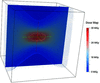Figure 1 Dose map of the Standard data collection described in §1.1(100 µm × 100 µm × 100 µm crystal, 20 µm × 20 µm FWHM beam, 90° wedge). The dark lines around the outside represent the edges of the crystal and the rotation axis is along the thin yellow line coming out of the plane of the page. The blue dotted isosurface represents the surface enclosing 95% of the absorbed dose (0.61 MGy), and the red dotted isosurface represents the Henderson limit of 20 MGy (Henderson, 1990). For this example, the region in the middle suffers a dose of 26.8 MGy, which is just below the 30 MGy experimental dose limit (Owen et al., 2006). The colour bar for the y-axis slice is shown to the right.

1.2. Aggregate dose metrics

In order to facilitate comparison of various data-collection strategies, aggregate metrics that indicate the dose absorbed across the crystal would be more appropriate than the standard and often ambiguous average dose' currently in common usage. The set suggested here are:

Average Dose, Whole Crystal (AD-WC). This is the average of the dose values for the whole crystal volume, i.e. the total absorbed energy over the total mass of the crystal. It is a crude metric that does not reflect the fact that the vast majority of the data may have been collected from one highly damaged region. Changes in beam shape or collection strategy for the same exposure time, beam energy and crystal composition should not result in large effects on this value since the total absorbed energy will be approximately constant. It is only meaningful if the crystal is smaller than the beam, and is then a good metric if the beam has a top-hat profile.

Average Dose, Exposed Region (AD-3FWHM). This is the average dose calculated with the same absorbed energy as for the AD-WC, but with the mass in the denominator of the dose equation calculated from the volume swept out by the beam. For the Gaussian beams described here, this is defined as the volume swept out by a rectangular mask of dimensions 3 × FWHM of the beam profile in x and y (which for a true Gaussian will include >99% of the flux and thus >99% of the absorbed energy).

95% Threshold Average Dose (TAD-95). This is the average dose for the region defined by the isodose surface which encloses 95% of the absorbed energy. This has the advantage of leaving out the very weakly exposed regions arising from the tails of the Gaussian beam profile, but the absolute value is clearly sensitive to the percentage chosen for the absorbed energy threshold.

Comparing these three average dose metrics, it is noted that there is a constant ratio between the numerators of the equations defining them, and all have similar values (1:0.99:0.95). The main difference between them is in the denominator of the dose equation (the mass term). For AD-WC, this is only a function of crystal size and density; for AD-3FWHM it is also a function of the number of FWHMs of the beam profile used for the threshold, and of any rotation or translation of the crystal during exposure; for TAD-95, it is a function of threshold chosen, the crystal size and density, the beam FWHM, flux and energy, and the rotation and translations of the crystal during the data collection.

Maximum Dose. This is the highest value of the dose reached anywhere in the crystal (i.e. voxel with highest dose). It is a useful metric since very high maximum doses can have a large negative impact on data quality and can significantly increase crystal non-isomorphism (Ravelli & McSweeney, 2000; Ravelli et al., 2002; Murray & Garman, 2002).

Dose Contrast. This is the ratio of the Maximum Dose to TAD-95 and is a useful quick metric which indicates the range of the dose across the region from which most of the diffraction has originated. Thus a lower rather than a high number implies that most of the data have come from a more homogeneously damaged region.

Dose Inefficiency. Minimizing the maximum dose is not a sufficient criterion by which to judge the effectiveness of a given data-collection strategy, since lowering the dose can trivially be achieved by reducing the exposure. A better metric to consider is the maximum dose per unit diffraction. Since, within the narrow energy range used in MX, the cross section for diffraction and the cross section for absorption events (and thus the absorbed energy) maintain a proportional relationship, the absorbed energy can be used as a proxy for the diffraction yield of the crystal. A Dose Inefficiency' metric can thus be defined as the maximum dose over the total absorbed energy (i.e. over the total diffracted intensity). The SI unit for this metric is kg−1, which reflects the fact that scaling a given crystal-strategy system up (larger crystal volume, higher total flux, unchanged flux density) will increase the amount of diffraction for the same maximum dose. This is intuitive since a bigger crystal illuminated with a bigger beam would be expected to diffract more than if both were smaller. A lower Dose Inefficiency implies that for each mJ absorbed the peak dose in the crystal is lower, and so the strategy is deemed better. Therefore halving the Dose Inefficiency means that the maximum dose is halved for a given amount of absorbed energy (and thus to first order, also for the same amount of diffraction).

For the typical experimental case described in §1.1, the results for these six metrics are presented in Table 2. The range of values obtained clearly illustrates how no single dose metric can describe the full picture of the effects induced by the dose distribution in the crystal. Different dose regions have different levels of unit-cell expansion (Ravelli & McSweeney, 2000; Murray & Garman, 2002; Ravelli et al., 2002), and when these regions are adjacent in a crystal (as in the case of a Gaussian beam) this will lead to strain along the crystal axes, which would in turn be expected to affect diffraction quality. It can thus be justifiably assumed that relatively lower Dose Contrast and Dose Inefficiency are indicators of better data-collection strategies. To achieve homogeneous dose distributions and thus minimize stresses building up within the crystal, the ideal beam from a radiation damage minimization point of view is a top-hat beam.

 Table 2Aggregate results for the simulation described in Table 1The definitions of the parameters are given in §1.2. Note the very large spread over the three dose metrics: 1:4.1:20.7 [Average (Whole Crystal):95% Threshold Average:Maximum Dose].
 Average Dose (Whole Crystal) 1.5 MGy 95% Threshold (1.11 MGy) Average Dose 6.0 MGy Maximum Dose 30.3 MGy Dose Contrast (Maximum/Threshold Average) 5.05 Total absorbed energy 1.86 mJ Dose Inefficiency (Maximum Dose/Absorbed Energy) 1.6 × 104 kg−1

Having highlighted the inadequate nature of a single average or peak dose for describing the absorbed energy profile for data collection performed with a Gaussian-shaped beam, the focus of what follows will be on using the above metrics to compare a number of methods for improving data quality by spreading the dose as evenly as possible throughout a crystal.

2. Methods

The simulation results presented in this paper were generated using Java code developed as part of an on-going major upgrade of the RADDOSE software code to give a new version: RADDOSE-3D (manuscript in preparation). This code effects a full voxel-based three-dimensional simulation of the dose profile of an MX experiment, and reports both three-dimensional dose fields and summary statistics. This is achieved by first creating a virtual representation of the crystal consisting of evenly spaced voxels at the specified resolution then and for each wedge: (i) determining, by rotation and translation operations, which of the crystal voxels intersect with the X-ray beam, (ii) for each affected voxel, calculating the specific X-ray flux at this point, as determined by the beam flux profile and the attenuation of the beam as it travels through parts of the crystal to reach the voxel, and (iii) calculating the absorbed energy at each voxel using this specific X-ray flux. The code finally generates summary statistics from the voxel map. For all the cases considered here, attenuation and absorption coefficients of μatt = 2.81 mm−1 and μabs = 2.37 mm−1, respectively, and a density of ρ = 1.2 g cm−3 were used. The μ values are based on an average empirical formulae taken from a survey of the Protein Data Bank (Berman et al., 2002) as used in a previous paper by Holton & Frankel (2010) and are for an incident X-ray energy of 12.4 keV. The density value is constituted from a combination of 50% each of a typical protein density (1.4 g cm−3) (Fischer et al., 2004) and of solvent [low-density amorphous ice, 0.94 g cm−3 (Mishima et al., 1985)]. All simulations were performed with 2 µm × 2 µm × 2 µm voxels and at an angular resolution of 1° per iteration, using an uncollimated Gaussian beam with various FWHM values. The details of the code and the method by which the calculations have been expedited will be presented elsewhere. A Visual Basic script was used to generate the large number of input data required to sample the parameter space, and the comparative plots were prepared using R (http://www.r-project.org/). The dose profiles were visualized using the Paraview software package (http://www.paraview.org/).

A total of 84 simulations were performed, using seven Gaussian beam FWHM sizes, four typical crystal shapes, and three established data-collection strategies. The crystal shapes were chosen to represent some of the more common crystal morphologies encountered by experimenters. Their dimensions were defined to give a constant volume across all shapes (Cube, 100 µm × 100 µm × 100 µm; Plate, 200 µm × 200 µm × 25 µm; Short Needle, 70 µm × 200 µm × 70 µm; Long Needle, 35.4 µm × 800 µm × 35.4 µm). Seven beams having equal total flux but different FWHM values in x and y (FWHM: 3 µm × 3 µm, 5 µm × 5 µm, 10 µm × 10 µm, 20 µm × 20 µm, 50 µm × 50 µm, 75 µm × 75 µm, 100 µm × 100 µm) were used in order to compare data-collection strategies for different crystal shapes. The three strategies compared were as follows:

Standard. 90° wedge collected symmetrically across one edge of the crystal with the beam centred for all dimensions of the crystal.

Helical. As for Standard, but translating the crystal along the goniometer axis during data collection. The translation was defined so that at the start and end positions the beam centre was half of its FWHM from the crystal edge. For example, the centre of a 10 µm × 10 µm beam on a Plate (200 µm × 200 µm × 25 µm) was translated from −95 µm to +95 µm (centre of crystal side being defined as 0 µm) along the goniometer axis, as shown in Fig. 2.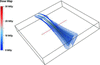Figure 2 Dose map for a Helical collection for a Plate crystal with a 10 µm × 10 µm FWHM beam. The outer isosurface (points) is a 0.71 MGy surface that encloses 95% of the absorbed energy. The inner surface is a 5 MGy surface for visual clarity.

Translate. As for Standard, except that the total exposure was broken up into several individual wedges at different positions on the crystal along the goniometer axis. The first wedge was collected half a beam profile FWHM from the edge of the crystal, then the crystal was translated by one beam profile FWHM for each subsequent wedge until the beam centre was within half a FWHM of the other edge of the crystal, as shown in Fig. 3for a Short Needle and a 20 µm × 20 µm beam for the simulations described above. A summary of the number of wedges for each beam size and crystal shape is included in the supplementary information as Table S1.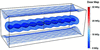Figure 3 Dose map of a Translational collection strategy for a Short Needle crystal. The outer isosurface (points) is a 0.11 MGy surface that encloses 95% of the absorbed energy. The intermediate and inner surfaces are presented for visual clarity at iso-values of 1.8 MGy and 2.5 MGy, respectively.

3. Results

Before analysing the results obtained for different data-collection strategies with each crystal type, the metrics must be compared in order to establish their relative merits, utility and relevance in evaluating the experimental protocols.

Figs. 4and 5show all the metrics plotted against the FWHM area of the beam profile for a Cube-shaped crystal subjected to a Standard strategy, and for a Long Needle with a Helical strategy, respectively. The beam FWHM area was chosen as an independent variable since, all other variables remaining constant, increasing the beam FWHM will have the effect of spreading the dose more evenly through the crystal and because, for a Gaussian beam, the peak intensity at constant flux decreases with increasing (FWHM)2. Looking first at the two most intuitive metrics, AD-WC and Maximum Dose, the behaviour is as expected. Since the AD-WC is only a function of absorbed energy, it is constant until the beam profile FWHM starts to be comparable with the crystal size, and then it begins to drop for both cases as more of the photons in the tails of the distribution are lost since they miss the crystal. For both of these strategies, the maximum dose is a proportional function of the peak intensity of the beam; it would thus be expected to fall as the beam peak intensity falls. As mentioned above, peak intensity goes as (FWHM)−2 for constant flux, and so on a log–log plot this follows a straight line with negative gradient.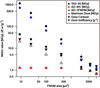Figure 4 Metric comparison for a Cube crystal with a Standard data collection. The y axis is shared by three units as indicated in the key. Note the log–log scale.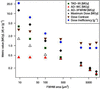Figure 5 Metric comparison for a Long Needle with a Helical strategy. The y axis is shared by three units as indicated in the key. Note the log–log scale.

Considering next the two other dose metrics for a Cube-shaped crystal, TAD-95 and AD-3FWHM, similar behaviour is observed for both the Standard–Cube and Helical–Long-Needle strategy–crystal-type conditions. They both track downwards as the mass in the dose equation increases with beam size, and then converge as the limit of including the whole crystal is approached. For the Standard–Cube case, the convergence occurs at around the 50 µm × 50 µm FWHM beam and, for the Helical–Long-Needle case, at around the 20 µm × 20 µm FWHM beam. This discrepancy is expected since the Long Needle has dimensions of 35.4 µm × 35.4 µm in the plane normal to the goniometer axis, and the Cube has dimensions of 100 µm × 100 µm in this plane. Thus the whole crystal will be irradiated by a 3×FWHM beam envelope for a smaller value of FWHM for the Long Needle, since the translation is performed along the whole length of the crystal during a Helical scan, making the length along the rotation axis irrespective to when full illumination is achieved.

Having considered all the dose metrics, TAD-95 was chosen as the preferred metric for use in evaluating strategies, since it has the advantage of being an average, and thus representative of more than just the peak dose. It also defines the mass in the dose equation intelligently, using an isosurface that encloses 95% of the total absorbed energy.

As a way of comparing the Maximum Dose worst-case' scenario returned by RADDOSE versions 1, 2 and 3 with the more representative TAD-95, Fig. 6shows these metrics plotted against each other for a Cube-shaped crystal. This is particularly instructive since Maximum Dose suggests very similar performance for Helical and Translational strategies, but, looking a little further, it is clear that a Translational strategy results in a lower dose than the Helical one in terms of TAD-95 for beams smaller than 75 µm × 75 µm FWHM. Similar trends are observed for the Plate, Short Needle and Long Needle, and are presented in the supplementary material (Figs. S1–S3).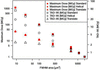Figure 6 Comparison of Maximum Dose and TAD-95 for a Cube crystal. The Maximum Dose appears not to differentiate between Helical and Translational strategies, although the TAD-95 is clearly lower for the Translational method. Note the log–log scale.

Whereas the dose metrics discussed above are informative concerning the average dose in different segmentations of the crystal, Dose Contrast and Dose Inefficiency offer a way of examining the dose distribution which is independent of the total exposure suffered by the crystal. Thus, doubling the exposure time in a collection protocol for a crystal would double the values of the dose metrics, but leave the Dose Contrast and Dose Inefficiency unchanged.

For both crystal-strategy pairs shown in Figs. 4and 5, the Dose Inefficiency (Maximum Dose/total absorbed energy) drops and then begins to level off for larger beams. This is caused by the absorbed-energy term being constant, and then dropping as a larger proportion of the beam is lost at the sides of the crystal (as seen in the AD-WC points). Consequentially, the tailing off is more pronounced for the Long Needle crystal which has smaller dimensions in the plane perpendicular to the goniometer axis. Dose Contrast also uses Maximum Dose as the numerator, but has TAD-95 as its denominator. Its behaviour for large beams is thus less affected by losing beam off the side of the crystals.

Although Dose Contrast offers an intuitive way of examining the spread of dose in a crystal, Dose Inefficiency is a more informative and complete metric, since it reports on the highest dose a crystal will experience for a given amount of diffraction: an immediately experimentally useful number.

Plots of the TAD-95 and Dose Inefficiency against beam FWHM for each of the crystal shapes (Cube, Plate, Short and Long Needles, respectively) are shown in Fig. 7. Looking first at the Cube, it can be noted that TAD-95 for the Translational strategy outperforms the Helical strategy, and both Translational and Helical outperform the Standard strategy. The conclusions relating to the Helical versus Standard data collection presented here are in agreement with experimental results to be found in the literature (Flot et al., 2010). The authors concluded that a helical data collection leads to a flatter profile in the inter-frame B-factors across the φ rotation range used (270°) relative to a Standard-type data collection. Their results were obtained for a 1.7 Å resolution dataset collected from a 10 µm × 300 µm × 10 µm crystal of the feruloyl esterase module of xylanase 10B from Clostridium thermocellum, using a beam with a FWHM of approximately 7.5 µm × 5 µm.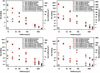Figure 7 (a) TAD-95/Dose Inefficiency plots for the four crystal shapes: (a) Cube, (b) Plate, (c) Short Needle and (d) Long Needle. In all four cases the Helical and Translational methods converge for both metrics at larger beam sizes. The crystals (c) and (d) have a longer dimension along the goniometer axis and exhibit a better Dose Inefficiency for smaller beams. Note log–log axes.

The Dose Inefficiency shows very similar performance for both the Translational and Helical dose-spreading strategies. This is because Dose Inefficiency reports on how well the Maximum Dose is minimized per unit diffraction, whereas TAD-95 reports on average dose. Thus both strategies are similarly effective in reducing the maximum dose per unit diffraction, but a translational scan spreads the bulk of the dose over a larger region, leading to a lower TAD-95. A similar effect, but with a smaller difference between Translational and Helical strategies, is observed for the Plate crystal.

For the Long and Short Needles, the same trend is observed in the TAD-95s, but the Dose Inefficiencies additionally begin to diverge, showing lower values for the Translational strategy. This is a small effect for the Short Needle, with a Helical:Translate ratio of 1.3 for a 3 µm × 3 µm FWHM beam. For the Long Needle the effect becomes much more pronounced with a ratio of 2.8 under the same conditions. This spread in the Dose Inefficiency can be understood as being due to the larger length of the crystal along the goniometer axis compared with the beam size. This results in the effect of the extra low-dose regions in a Translational strategy over a Helical strategy being compounded, leading to a lower maximum dose at a given total exposure for the Translational strategy.

It is instructive to note that for all four crystal shapes the Dose Contrast shows the opposite trend: a lower dose contrast for a Helical scan (Cube shown in Fig. 8, and the other three crystal shapes shown as Figs. S4–S6 in the supplementary information). This is because a translation of the crystal divides the peak dose by the number of possible steps which will avoid excessive overlap of the beam positions: the more steps, the greater the reduction. In terms of peak dose, a Helical strategy is the N → ∞ limit of this. This serves as a good illustration of why Dose Inefficiency is a superior metric to Dose Contrast: the better' dose contrast for a helical scan is simply caused by the higher maximum dose at N points in the crystal and does not reflect the improvement found in other regions through using a Translational strategy.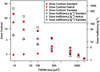Figure 8 Comparison of Dose Contrast and Dose Inefficiency metrics. Contrast is lower for Helical than for Translational strategies, despite similar Dose Inefficiencies, illustrating the shortcomings of using Dose Contrast as the main metric. Note log–log axes.

4. Discussion

The central question raised by these results is how to interpret them in terms of day-to-day experimental practice. Current guidelines exist in the form of the 20 MGy dose limit predicted for MX by Henderson (1990) and a 30 MGy experimental dose limit which provides a recommendation regarding the dose beyond which a crystal is likely to give data of questionable biological fidelity (Owen et al., 2006). This limit was measured under experimental conditions carefully optimized to be as close to the even-dose case as possible. Indeed, researchers working to understand radiation damage in MX make significant efforts to ensure that these conditions are well emulated in their experiments. For instance, this is achieved through experimental protocols such as matching the crystal size to the beam and ensuring a top-hat beam profile for data collection (Murray & Garman, 2002; Owen et al., 2006), or by limiting the oscillation of the crystals to small wedges (Kmetko et al., 2006), so that the irradiated region does not suffer from an uneven dose distribution such as the one shown in Fig. 1.

In order to interpret a dose map in the context of existing guidelines, the physics behind diffraction pattern formation must be considered. Each elastically scattered photon will have originated from a region of the crystal in some state of damage ranging from a zero-dose state to a highly damaged state which will have absorbed a dose well above 30 MGy. It can be hypothesized that the final diffraction pattern is a linear superposition of the individual patterns that would originate from each of these damage states. The experimental dose limit indicates that a region of crystal that has suffered a dose above 30 MGy may have compromised biological fidelity. Experimentalists should therefore aim to minimize or, ideally, eliminate any regions at or above that limit while simultaneously extracting the maximum amount of diffraction from a crystal. The Dose Inefficiency metric is a way of describing how well a proposed strategy achieves this goal. TAD-95, on the other hand, is a metric which indicates the average final dose state of the crystal. Strategies can be optimized to minimize Dose Inefficiency and experiments can be monitored via TAD-95. These then give useful practical dose metrics, since the dose field from each simulation result is interpretable as a single number which can be compared across different experimental scenarios.

The most pronounced result that can be surmised from Fig. 7is that the largest beam size (100 µm × 100 µm FWHM) is the most effective at minimizing Dose Inefficiency for all the cases considered, and also produces a lower TAD-95 for the same total flux. Across the board, matching the beam size to the crystal is the most efficient way of exposing a crystal evenly. Given a crystal shape and beam size (or relative beam size in the case of crystals that are not of similar size to the ones used as examples here), Fig. 7can be used to evaluate the comparative advantages of a Helical or a Translational strategy over a Standard data collection. Fig. 7can thus be used as a guide to evaluate whether the advantage is worth the extra complexity of these data-collection protocols.

An additional strategy that has been reported is to use a beam size that matches the crystal in the vertical axis and to then perform a helical scan. An additional simulation was carried out for a 100 µm × 10 µm beam using a Helical strategy on the Cube crystal. When compared with a 100 µm × 100 µm beam, the Dose Inefficiencies were almost identical: 1.2 g−1 for the Standard 100 µm × 100 µm beam and 1.1 g−1 for the Helical 100 µm × 10 µm beam. Since the crystals in the two simulations absorbed different amounts of energy (and hence diffracted different numbers of photons), for comparison the TAD-95 was also normalized. This again gave very similar results: 0.80 g−1 for the Standard 100 µm × 100 µm beam and 0.84 g−1 for the Helical 100 µm × 10 µm beam. These simulations show that dose distributions can be optimized by matching only the vertical axis of the beam to the crystal size and employing a Helical strategy.

Clearly, for a full simulation of real-life MX data collections, the photon flux density and beam shape are required. Importantly, it will also be necessary to have accurate information on the crystal topology, since crystals do not necessarily grow in the shapes modelled above. The ability to parameterize crystal shapes in situ on the beamline is an active area of research (see, for instance, Brockhauser et al. 2008; Khan et al., 2012). A further issue still to be addressed is RADDOSE versions and also RADDOSE-3D do not take into account the possibility of the escape of the primary photoelectrons from the crystal surface. Their range for 15.1 and 18.5 keV incident X-rays has been found to be around 4 µm (Sanishvili et al., 2011) experimentally. Simulations show that this phenomenon can reduce the dose suffered by microcrystals, especially if using beams with energies above 25 keV (Nave & Hill, 2005; Cowan & Nave, 2008). For RADDOSE-3D to have better applicability for microcrystals and nanocrystals [such as those used in serial femtosecond MX crystallography (e.g. Chapman et al., 2011)], the photoelectron escape is clearly an important addition to be made to the dose description.

5. Conclusions

The relative effectiveness of two dose-spreading strategies compared with a non-translational data-collection strategy has been evaluated here using two new metrics, the Threshold Average Dose and the Dose Inefficiency. Our simulations show an order-of-magnitude reduction in Dose Inefficiency for either a Helical or Translational strategy, and the results can be used by the experimenter to judge when either of these strategies would be worth employing on-line.

The profile of the beam has a pivotal effect on the dose distribution within an irradiated crystal, and top-hat-shaped beams will always result in more uniform dose distributions than Gaussian beams.

In terms of minimizing the maximum dose per unit diffraction, the most efficient way of collecting data with a Gaussian beam is to closely match the beam size to the crystal in order to obtain the most even dose profile possible while not excessively exposing the non-crystal regions of the loop (cryobuffer/mother-liquor). As already discussed, this effect can also be achieved by matching only the vertical (perpendicular to the rotation axis) size of the beam to the crystal dimensions and performing a Helical scan. This has an important implication for beamline design, since it only requires collimation in one dimension to optimize dose distribution. Relative to collimating in two dimensions, this would result in a linear reduction in the amount of beam area lost to the collimator for a given Cube crystal smaller than the vertical beam dimensions.

When this beam shape is not available, either a Helical or a Translational strategy provides an improvement in both the TAD-95 and the Dose Inefficiency. Judged by these metrics, a Translational strategy is more effective than a Helical one, and this is more pronounced the smaller the beam is relative to the crystal. Since these results are limited to the metrics discussed here, other effects, such as the implications for scaling data collected using a Helical or Translational strategy, could affect these conclusions in experimental reality, and must thus also be considered for a more complete treatment.

This paper has highlighted the large improvement in dose homogeneity that could be obtained by optimizing the data-collection protocols for a particular beam and crystal size. Clearly, there is a need for experimental investigations into the impact of strategies with different Dose Inefficiencies on final data quality and this will be a focus of future work.

Footnotes

1Supplementary data for this paper are available from the IUCr electronic archives (Reference: XH5033). Services for accessing these data are described at the back of the journal.

Acknowledgements

We gratefully acknowledge the UK's Engineering and Physical Sciences Research Council (EPSRC) for funding, through studentships at the Life Science Interface (OZ) and the Systems Biology (MG) programmes at the University of Oxford's Doctoral Training Centre. We also thank James Holton and Robert Fischetti for valuable and insightful conversations on the subject of dose metrics and distributions. Finally, we would like to thank the referees for their highly constructive comments which have benefited the final manuscript.

ReferencesJOURNAL OFSYNCHROTRONRADIATION